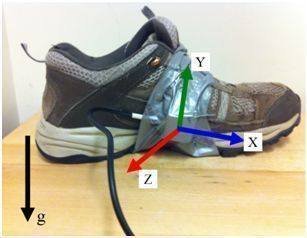# Acceleration DataAccelerometer fixed to shoe.

Designing the nonlinear energy harvester involved several steps. The first step was to record the acceleration of a person while walking. Below are the raw data files of acceleration signals recorded for different human motions. The data files with 3 directions were recorded using a Vernier tri-axial 5-g accelerometer. The data files with 1 direction were recorded using a Vernier 25-g uni-axial accelerometer. These data files are formatted for Matlab.

Here is a Matlab file to format the raw data, obtain its FFT, and plot the resulting time series and FFT of the acceleration, velocity, and displacement. You might want to adjust line 44 to zero out constant or very low frequency terms, which may cause drift in the displacement plots. You might also want to adjust line 104 to vary how many FFT components are plotted.

 Body location Motion type File Notes Hip Walk Open Columns are [time; y'']. Hip Walk quickly Open Columns are [time; y'']. Hip Run Open Columns are [time; y'']. Foot Walk Open Columns are [time; x''; y''; z'']; corresponding to Figure 1. Foot Run, forward Open Columns are [time; x'']; corresponding to Figure 1. Foot Run, up Open Columns are [time; y'']; corresponding to Figure 1.